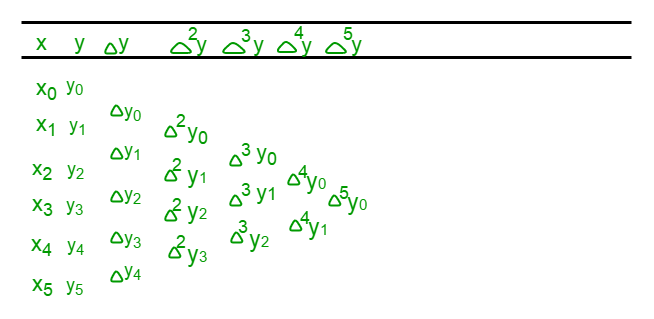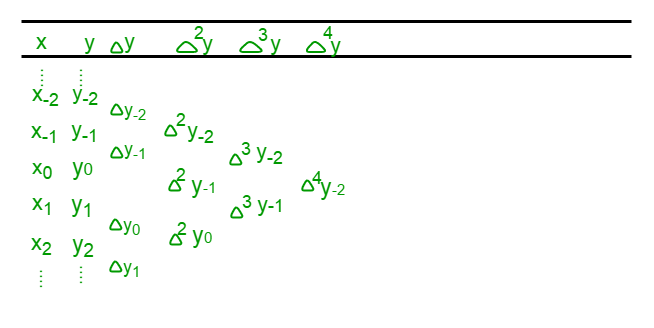Related Articles

# Program for Stirling Interpolation Formula

• Difficulty Level : Hard
• Last Updated : 04 Jan, 2019

Given n number of floating values x, and their corresponding functional values f(x), estimate the value of the mathematical function for any intermediate value of the independent variable x, i.e., at x = a.
Examples:

Input : n = 5
x= 0, x= 0.5,
x= 1.0, x= 1.5,
x= 2.0
f(x) = 0, f(x) = 0.191,
f(x) = 0.341, f(x) = 0.433,
f(x) = 0.477
a = 1.22
Output : The value of function at 1.22 is 0.389 .
As can be seen f(1.0) = 0.341 and f(1.5) = 0.433,
so f(1.22) should be somewhere in between these
two values . Using Stirling Approximation, f(1.22)
comes out to be 0.389.

Input : n = 7
x= 0, x= 5,
x= 10, x= 15,
x= 20, x= 25,
x= 30
f(x) = 0, f(x) = 0.0875,
f(x) = 0.1763, f(x) = 0.2679,
f(x) = 0.364, f(x) = 0.4663,
f(x) = 0.5774
a = 16
Output : The value of function at 16 is 0.2866 .


## Recommended: Please try your approach on {IDE} first, before moving on to the solution.

Stirling Interploation

Stirling Approximation or Stirling Interpolation Formula is an interpolation technique, which is used to obtain the value of a function at an intermediate point within the range of a discrete set of known data points .

Stirling Formula is obtained by taking the average or mean of the Gauss Forward and Gauss Backward Formula . Both the Gauss Forward and Backward formula are formulas for obtaining the value of the function near the middle of the tabulated set .

How to find

Stirling Approximation involves the use of forward difference table, which can be prepared from the given set of x and f(x) or y as given below –This table is prepared with the help of x and its corresponding f(x) or y . Then, each of the next column values is computed by calculating the difference between its preceeding and succeeding values in the previous column, like= y– y,= y– y,=, and so on.

Now, the Gauss Forward Formula for obtaining f(x) or y at a is:where,
p =,
a is the point where we have to determine f(x), xis the selected value from the given x which is closer to a (generally, a value from the middle of the table is selected), and h is the difference between any two consecutive x. Now, ybecomes the value corresponding to xand values before xhave negative subscript and those afterhave positive subscript, as shown in the table below –And the Gauss Backward Formula for obtaining f(x) or y at a is :Now, taking the mean of the above two formulas and obtaining the formula for Stirling Approximation as given below –Here, q is the same as p in Gauss formulas and rest all symbols are the same.

When To Use

• Stirling Approximation is useful when q lies betweenand• Outside this range, it can still be used, but the accuracy of the computed value would be less.
• It gives the best estimate for the range< q <.

## C++

 // C++ program to demonstrate Stirling// Approximation#include using namespace std;  // Function to calculate value using // Stirling formulavoid Stirling(float x[], float fx[], float x1,                                    int n){    float h, a, u, y1 = 0, N1 = 1, d = 1,    N2 = 1, d2 = 1, temp1 = 1, temp2 = 1,    k = 1, l = 1, delta[n][n];        int i, j, s;    h = x - x;    s = floor(n / 2);    a = x[s];    u = (x1 - a) / h;      // Preparing the forward difference    // table    for (i = 0; i < n - 1; ++i) {        delta[i] = fx[i + 1] - fx[i];    }    for (i = 1; i < n - 1; ++i) {        for (j = 0; j < n - i - 1; ++j) {            delta[j][i] = delta[j + 1][i - 1]                          - delta[j][i - 1];        }    }      // Calculating f(x) using the Stirling     // formula    y1 = fx[s];      for (i = 1; i <= n - 1; ++i) {        if (i % 2 != 0) {            if (k != 2) {                temp1 *= (pow(u, k) -                           pow((k - 1), 2));            }             else {                temp1 *= (pow(u, 2) -                           pow((k - 1), 2));            }            ++k;            d *= i;            s = floor((n - i) / 2);            y1 += (temp1 / (2 * d)) *                    (delta[s][i - 1] +                    delta[s - 1][i - 1]);        }         else {            temp2 *= (pow(u, 2) -                       pow((l - 1), 2));            ++l;            d *= i;            s = floor((n - i) / 2);            y1 += (temp2 / (d)) *                  (delta[s][i - 1]);        }    }      cout << y1;}  // Driver main functionint main(){    int n;    n = 5;    float x[] = { 0, 0.5, 1.0, 1.5, 2.0 };    float fx[] = { 0, 0.191, 0.341, 0.433,                             0.477 };      // Value to calculate f(x)    float x1 = 1.22;      Stirling(x, fx, x1, n);    return 0;}

## Java

 // Java program to demonstrate Stirling // Approximationimport java.io.*;import static java.lang.Math.*;  public class A {    static void Stirling(double x[], double fx[],                         double x1, int n)    {        double h, a, u, y1 = 0, N1 = 1, d = 1,            N2 = 1, d2 = 1, temp1 = 1,          temp2 = 1, k = 1, l = 1, delta[][];                  delta = new double[n][n];        int i, j, s;        h = x - x;        s = (int)floor(n / 2);        a = x[s];        u = (x1 - a) / h;          // Preparing the forward difference        // table        for (i = 0; i < n - 1; ++i) {            delta[i] = fx[i + 1] - fx[i];        }        for (i = 1; i < n - 1; ++i) {            for (j = 0; j < n - i - 1; ++j) {                delta[j][i] = delta[j + 1][i - 1]                               - delta[j][i - 1];            }        }          // Calculating f(x) using the Stirling         // formula        y1 = fx[s];          for (i = 1; i <= n - 1; ++i) {            if (i % 2 != 0) {                if (k != 2) {                    temp1 *= (pow(u, k) -                               pow((k - 1), 2));                }                 else {                    temp1 *= (pow(u, 2) -                               pow((k - 1), 2));                }                ++k;                d *= i;                s = (int)floor((n - i) / 2);                y1 += (temp1 / (2 * d)) *                      (delta[s][i - 1] +                      delta[s - 1][i - 1]);            }             else {                temp2 *= (pow(u, 2) -                        pow((l - 1), 2));                ++l;                d *= i;                s = (int)floor((n - i) / 2);                y1 += (temp2 / (d)) *                      (delta[s][i - 1]);            }        }          System.out.print(+ y1);    }      // Driver main functionpublic static void main(String args[])    {        int n;        n = 5;        double x[] = {0, 0.5, 1.0, 1.5, 2.0 };        double fx[] = {0, 0.191, 0.341, 0.433,                                      0.477 };          // Value to calculate f(x)        double x1 = 1.22;          Stirling(x, fx, x1, n);    }}

## Python3

 # Python3 program to demonstrate Stirling# Approximationimport math  # Function to calculate value using # Stirling formuladef Stirling(x, fx, x1, n):      y1 = 0; N1 = 1; d = 1;    N2 = 1; d2 = 1;     temp1 = 1; temp2 = 1;    k = 1; l = 1;     delta = [[0 for i in range(n)]                for j in range(n)];      h = x - x;    s = math.floor(n / 2);    a = x[s];    u = (x1 - a) / h;      # Preparing the forward difference    # table    for i in range(n - 1):         delta[i] = fx[i + 1] - fx[i];    for i in range(1, n - 1):        for j in range(n - i - 1):            delta[j][i] = (delta[j + 1][i - 1] -                            delta[j][i - 1]);      # Calculating f(x) using the Stirling formula    y1 = fx[s];      for i in range(1, n):        if (i % 2 != 0):             if (k != 2):                 temp1 *= (pow(u, k) - pow((k - 1), 2));            else:                temp1 *= (pow(u, 2) - pow((k - 1), 2));            k += 1;            d *= i;            s = math.floor((n - i) / 2);            y1 += (temp1 / (2 * d)) * (delta[s][i - 1] +                                        delta[s - 1][i - 1]);        else:            temp2 *= (pow(u, 2) - pow((l - 1), 2));            l += 1;            d *= i;            s = math.floor((n - i) / 2);            y1 += (temp2 / (d)) * (delta[s][i - 1]);      print(round(y1, 3));  # Driver Coden = 5;x = [0, 0.5, 1.0, 1.5, 2.0 ];fx = [ 0, 0.191, 0.341, 0.433, 0.477];  # Value to calculate f(x)x1 = 1.22;Stirling(x, fx, x1, n);  # This code is contributed by mits

## C#

 // C# program to demonstrate Stirling // Approximationusing System;  public class A {static void Stirling(double[] x, double[] fx,                     double x1, int n){    double h, a, u, y1 = 0, d = 1, temp1 = 1,                     temp2 = 1, k = 1, l = 1;    double[,] delta;          delta = new double[n, n];    int i, j, s;    h = x - x;    s = (int)Math.Floor((double)(n / 2));    a = x[s];    u = (x1 - a) / h;      // Preparing the forward difference     // table    for (i = 0; i < n - 1; ++i)     {        delta[i, 0] = fx[i + 1] - fx[i];    }    for (i = 1; i < n - 1; ++i)     {        for (j = 0; j < n - i - 1; ++j)         {            delta[j, i] = delta[j + 1, i - 1]                         - delta[j, i - 1];        }    }      // Calculating f(x) using the Stirling     // formula    y1 = fx[s];      for (i = 1; i <= n - 1; ++i)     {        if (i % 2 != 0)         {            if (k != 2)             {                temp1 *= (Math.Pow(u, k) -                           Math.Pow((k - 1), 2));            }             else             {                temp1 *= (Math.Pow(u, 2) -                           Math.Pow((k - 1), 2));            }            ++k;            d *= i;            s = (int)Math.Floor((double)((n - i) / 2));            y1 += (temp1 / (2 * d)) *                   (delta[s, i - 1] +                   delta[s - 1, i - 1]);        }         else         {            temp2 *= (Math.Pow(u, 2) -                      Math.Pow((l - 1), 2));            ++l;            d *= i;            s = (int)Math.Floor((double)((n - i) / 2));            y1 += (temp2 / (d)) *                  (delta[s, i - 1]);        }    }      Console.Write(+ y1);}  // Driver Codepublic static void Main(){    int n;    n = 5;    double[] x = {0, 0.5, 1.0, 1.5, 2.0 };    double[] fx = {0, 0.191, 0.341, 0.433,                                0.477 };      // Value to calculate f(x)    double x1 = 1.22;      Stirling(x, fx, x1, n);}}  // This code is contributed // by Akanksha Rai

## PHP

 

Output:
0.389


The main advantage of Stirling’s formula over other similar formulas is that it decreases much more rapidly than other difference formula hence considering first few number of terms itself will give better accuracy, whereas it suffers from a disadvantage that for Stirling approximation to be applicable there should be a uniform difference between any two consecutive x.

Reference – Higher Engineering Mathematics by B.S. Grewal.

This article is contributed by Mrigendra Singh. If you like GeeksforGeeks and would like to contribute, you can also write an article using contribute.geeksforgeeks.org or mail your article to contribute@geeksforgeeks.org. See your article appearing on the GeeksforGeeks main page and help other Geeks.